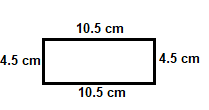# Kerala Syllabus 7th Standard Maths Solutions Chapter 9 Ratio

## Kerala State Syllabus 7th Standard Maths Solutions Chapter 9 Ratio

### Ratio Text Book Questions and Answers

Same shape Textbook Page No. 116

In both the rectangles below, the length is 1 centimetre more than the breadth.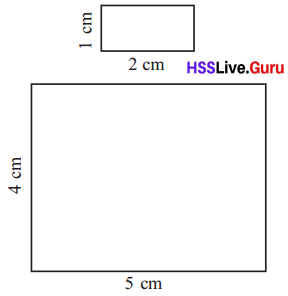But not only the length of these are different, they look different also. In the larger rectangle, the sides look almost the same. Draw a rectangle of length 50 centimetres and breadth 49 centimetres in a larger sheet of paper. It looks almost a square, doesn’t it?
In the first rectangle above, length is double the breadth. Now look at this rectangle.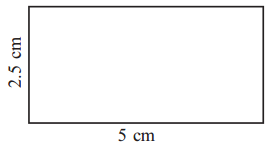Again the length is double the breadth. Even though it is larger than the first, they have the same shape, right?
As the length is double the breadth in the second rectangle. The first and the second rectangle will have the same shape.Changing scale

Look at this photo:The shorter side is 2 centimetres and the longer side, 3 centimetres; that is, the longer side is 1$$\frac{1}{2}$$ times shorter side.
Suppose we make the shorter side 3 centimetres and longer side 4.5 centimetres.Still, the longer side is 1$$\frac{1}{2}$$ times the shorter side.
Now suppose we change the shorter side to 3 centimetres and increase the longer side also by 1 centimetre, making it 4 centimeters.Does the picture look right?
Yes, the picture looks right.
Suppose if we change the shorter side to 3 centimetres and the longer side to 4 centimetres. The longer side is 1$$\frac{1}{3}$$ times the shorter side.

TV Math

The sizes of TV sets are usually given as 14 inch, 17 inch, 20 inch and so on. What does it mean?
The TV screen is a rectangle: and these are lengths of the diagonals of the screen.
Does it determine the size of the screen? Rectangles of different width and lengths can have the same diagonal:In the modern TV sets, the ratio of length to width is 16 : 9. In the earlier days, it was 4 : 3.
See their difference in two TV screens of the same diagonal length.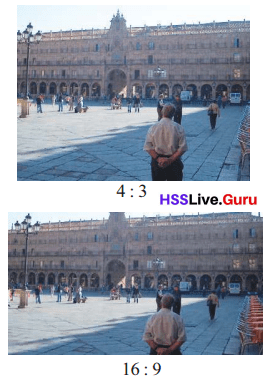Flags Textbook Page No. 119

When we draw our National Flag, not only should the colours be right, the ratio of width to length should also be correct. This ratio is 2 : 3.
That is, in drawing our flag, if the length is taken as 3 centimetres, the width should be 2 centimetres.This ratio is different for flags of other countries. For example, in the flag of Australia, this ratio is 1 : 2.And in the flag of Germany, it is 3 : 5.Without fractions Textbook Page No. 120

When quantities like length are measured using a definite unit, we may not always get counting numbers; and it is this fact which led to the idea of fractions.

In comparing the sizes of two quantities, one question is whether both can be given as counting numbers, using a suitably small unit of measurement. It is this question that leads to the idea of ratio.

For example, suppose the length of two objects are found as $$\frac{2}{5}$$ and $$\frac{3}{5}$$, when measured using a string. If $$\frac{1}{5}$$ of the string is taken as the unit, we can say the length of the first is 2 and that of the second is 3. This is the meaning of
saying the ratio of the lengths is 2 : 3.
Suppose the length of two objects are $$\frac{1}{3}$$ and $$\frac{1}{5}$$ of the string.
To get both lengths as counting numbers, what fraction of the string can be taken as a unit of measurement?

Circle relations

Look at the pieces of circles below: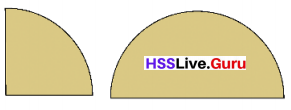The smaller piece is $$\frac{1}{4}$$ of the circle and the larger piece is $$\frac{1}{2}$$ of the circle.
So, the larger piece is twice the size of the smaller. That is, the ratio of the sizes of small and large pieces is 1 : 2.
Now look at these pieces: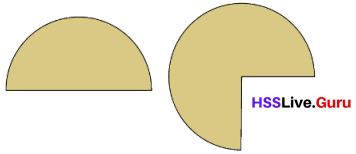What is the ratio of their sizes?
Let’s measure each using $$\frac{1}{4}$$ of circles.
The smaller figure has two such pieces. What about the larger?
Let’s measure each using $$\frac{1}{4}$$ of circles.
The larger figure has three such pieces.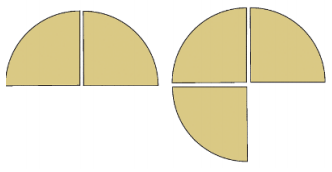So what is the ratio of the sizes of these two?
Let’s measure each using $$\frac{1}{4}$$ of circles.
The smaller piece has two such pieces and the larger piece has three such pieces.
2×$$\frac{1}{4}$$ : 3×$$\frac{1}{4}$$
$$\frac{2}{4}$$ : $$\frac{3}{4}$$
Therefore, the ratio will be 2 : 3Moving Ratio Textbook Page No. 122
Have you taken apart toy cars or old clocks? There are many toothed wheels in such mechanisms. See this picture:It is a part of a machine. In the two whole wheels we see, the smaller has 13 teeth and the larger has 21. So during the time that the smaller circle makes 21 revolutions, the larger one would have made only 13 revolutions.
The speed of rotation of machines is controlled by arranging such wheels with different number of teeth.

Sand and Cement

In construction of a building, sand and cement are used in definite ratios, but the ratios are different depending on the purpose. When one bowl of cement and five bowls of sand are mixed, the ratio of cement to sand is 1 : 5

When one sack of cement is mixed with five sacks of sand, the ratio is the same. But to set a brick wall, this much cement may not be needed. In this case, the ratio may be 1 : 10 or 1 : 12.

Ratio of Parts Textbook Page No. 124

We can use ratio to compare parts of a whole also. For example, in the picture below, the lighter part is $$\frac{3}{8}$$ of the circle, and the darker part is $$\frac{5}{8}$$ of the circle.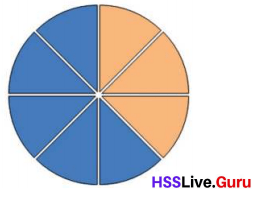These two parts together make up the whole circle. The ratio of the sizes of these parts is 3 : 5.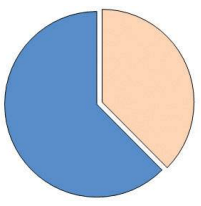So here, the ratio 3 : 5 indicate the two fractions $$\frac{3}{8}$$ and $$\frac{5}{8}$$.

Generally in such instances, a ratio of the numbers indicates fractions of equal denominators adding up to 1.

Meaning of ratio Textbook Page No. 125

If we know only the ratio of two quantities, we can’t say exactly how much they are; but they can be compared in several ways.
For examples, suppose the volumes of two pots are said to be in the ratio 2 : 3. We can interpret this in the following ways:

• To fill the smaller pot, we need $$\frac{2}{3}$$ of the larger pot.
• To fill the bigger pot, we need $$\frac{3}{2}$$ = 1$$\frac{1}{2}$$ times the smaller pot.
• Whether we take $$\frac{1}{2}$$ of the water in the smaller pot, or $$\frac{1}{3}$$ of the water in the larger pot, we get the same amount of water.
• If we fill both pots and pour them into a large pot, $$\frac{2}{5}$$ of the total amount of water
is from the smaller pot and $$\frac{3}{5}$$ from the larger.

If the length of two pieces of rope are said to be in the ratio 3 : 5, what all interpretations like this can you make?
If the length of two pieces of rope is 3 : 5.Then the following interpretations can be made.
The smaller rope is $$\frac{3}{5}$$ of the length of the larger rope.
The smaller rope will be $$\frac{3}{8}$$ of the total rope length.

Three measures

Look at this triangle:In it, the longest side is double the shortest. The side of medium length is one and a half times the shortest.
Saying this with ratios, the lengths of the shortest and the longest sides are in the ratio 1 : 2 and those of the shortest and the medium are in the ratio 2 : 3.
What is the ratio of the lengths of the medium side and the longest side?
We can say this in another way: if we use a string of length 1.5 centimetres, the shortest side is 2, the medium side is 3 and the longest side is 4.
The can be shortened by saying the sides are in the ratio 2 : 3 : 4.

Triangle Math Textbook Page No. 127

How many triangles are there with ratio of sides 2 : 3 : 4?
The lengths of sides can be 2 cm, 3 cm, 4cm.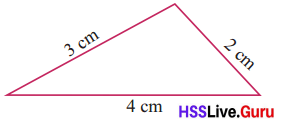Or they can be 1 cm, 1.5 cm, 2 cmWe can take metres instead of centimetres.
Thus we have several such triangles.
In all these, what fraction of the perimeter is the shortest side?
And the side of medium length?
The longest side?
The perimeter of a triangle is 80 centimetres and its sides are in the ratio 5 : 7 : 8. Can you compute the actual lengths of sides?
Let the ratio be x.Then the sides will be 5x, 7x and 8x.
Given that perimeter is 80 cm.
5x + 7x + 8x = 80
20x = 80
x = 4
Since the value of x is 4, the lengths will be 5×4=20cm, 7×4=28cm and 8×4=32cm.

What if the perimeter is 1 metre?
1 metre equals 100 centimetres.
Let the ratio be x.Then the sides will be 5x, 7x and 8x.
Given that perimeter is 100 cm.
5x + 7x + 8x = 100
20x = 100
x = 5
Since the value of x is 5, the lengths will be 5×5=25cm, 7×5=35cm and 8×5=40cm.

Length and width Textbook Page No. 116

Look at these rectangles:Is there any common relation between the lengths and widths of all these?
In all these, the length is twice the width, right? (We can also say width is half the length)
In the language of mathematics, we state this fact like this:
In all these rectangles, the width and length are in the ratio one to two.
In writing, we shorten the phrase “one to two” as 1 : 2; that is
In all these rectangles, the width and length are in the ratio 1 : 2.

In the rectangle of width 1 centimetre and length 2 centimetres, the length is twice the width. In a rectangle of width 1 metre and length 2 metres also, we have the same relation. So, in both these rectangles, width and length are in the ratio one to two (1 : 2).
We can state this in reverse: in all these rectangles, length and width are in the ratio two to one (2 : 1).
What is the width to length ratio of the rectangle below?The length of the given rectangle is 6cm and width is 2cm.
Therefore, the width to length ratio for the above rectangle will be 1 : 3.

The length of the given rectangle is 4.5cm and width is 1.5cm.
Therefore, the width to length ratio for the above rectangle will be 1 : 3.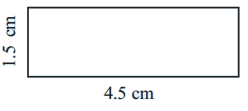In both, the length is 3 times the width. So what is the ratio of the width to the length?
In the above two rectangles, the length is thrice the width.
The ratio of the width to the length is 1 : 3.

How do we say this as a ratio?
The width and length are in the ratio one to three.

1 metre means loo centimetres. So in such a rectangle, the ratio of the width to the length is 1: 50.
Now look at these rectangles:In both, the length is one and a half times the width.
How do we say this as a ratio?
We can say one to one and a half; but usually we avoid fractions when we state ratios.
Suppose we take the width as 2 centimetres.
What is 1$$\frac{1}{2}$$ times 2?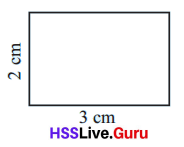So, we say that in rectangles of this type, the ratio of width to length is two to three and write 2 : 3.
Can’t we say here that the ratio is 4 : 6 ?
Nothing wrong in it; but usually ratios are stated using the least possible counting numbers.
So how do we state using ratios, the fact that the length of a rectangle is two and a half times the width?

If the width is 1 centimetre, then the length is 2$$\frac{1}{2}$$ centimetres.
What if the width is 2 centimetres?
Length would be 5 centimetres.
So, we say that width and length are in the ratio 2 to 5.

What if the length is one and a quarter times the width?
If the width is 1 centimetre, the length is 1$$\frac{1}{4}$$ centimetre.
If the width is 2 centimetres then the length is 2$$\frac{1}{2}$$ centimetres.
Still we haven’t got rid of fractions.
Now if width is 4 centimetres, what would be the length?

So, in all such rectangles, the width and length are in the ratio 4 : 5.
Do you notice another thing in all these?
If we stretch or shrink both width and length by the same factor, the ratio is not changed. For example, look at this table.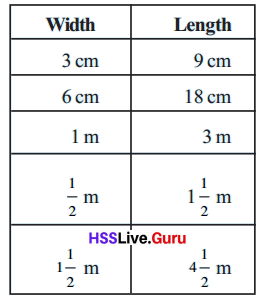In all these, the length is 3 times the width; or the reverse, the length is $$\frac{1}{3}$$ of the width.
In terms of ratio, we say width and length are in the ratio 1 : 3 or length and width are in the ratio 3 : 1.
In terms of ratio, we say width and length are in the ratio 1: 3 or length and width are in the ratio 3: 1.

• For all rectangles of dimensions given below, state the ratio of width to length, using the least possible counting numbers:

• width 8 centimetres, length 10 centimetres
The simplest form for the given numbers 8:10 is 4 : 5
Therefore, the ratio of width to length will be 4 : 5

• width 8 metres length 12 metres
The simplest form for the given numbers 8:12 is 4 : 3
Therefore, the ratio of width to length will be 2 : 3

• width 20 centimetres length 1 metre
The given numbers cannot be written in simplest form.
Therefore, the ratio of width to length will be 20 : 1

• width 40 centimetres length 1 metre
The given numbers cannot be written in simplest form.
Therefore, the ratio of width to length will be 40 : 1

• width 1.5 centimetres length 2 centimetres
The simplest form for the given numbers 1.5:2 is 3 : 4
Therefore, the ratio of width to length will be 3 : 4

• In the table below two of the width, length and their ratio of some rectangles are given. Calculate the third and fill up the table.• What does it mean to say that the width to length ratio of a rectangle is 1: 1 ? What sort of a rectangle is it?
The width to length ratio of a rectangle is 1 : 1.
Here the width of the rectangle and length of the rectangle is same.
If the width and the length of rectangle is same, then it is a square.Other quantities Textbook Page No. 120There are two ropes, the shorter $$\frac{1}{3}$$ metre long and the longer, $$\frac{1}{2}$$ metre long. What is the ratio of their lengths?

We can do this in different ways.
We can check how much times $$\frac{1}{3}$$ is $$\frac{1}{2}$$.
$$\frac{1}{2}$$ ÷ $$\frac{1}{2}$$ = $$\frac{3}{2}$$
So, the length of the longer rope is $$\frac{3}{2}$$ times that of the shorter.
That is, 1$$\frac{1}{2}$$ times.
If the length of the shorter rope is taken as 1, the length of the longer is 1$$\frac{1}{2}$$; if 2, then 3.
So the length of the shorter and longer rope are in the ratio 2 : 3.

We can also think in another manner. As in the case of the width and length of rectangles, we can imagine the length to be stretched by the same factor; and the ratio won’t change.
Suppose we double the length of each piece of rope.
Then the length of the shorter is $$\frac{2}{3}$$ metres and that of the longer 1 metre. This doesn’t remove fractions.

By what factor should we stretch to get rid of fractions? How about 6 ?
6 times $$\frac{1}{3}$$ is 2.
6 times $$\frac{1}{2}$$ is 3.
The shorter is now 2 metres and the longer is 3 metres. So the ratio is 2 : 3.
There is yet another way; we can write
$$\frac{1}{3}$$ = $$\frac{2}{6}$$ $$\frac{1}{2}$$ = $$\frac{3}{6}$$
That is, we can think of the shorter rope as made up of 2 pieces of $$\frac{1}{6}$$ metres each and the longer one as 3 of the same $$\frac{1}{6}$$ metres. In this way also, we can calculate the ratio as 2 to 3.
Now look at this problem : to fill a can we need only half the water in a bottle.

To fill a larger can, we need three quarters of the bottle. What is the ratio of the volume of the smaller can to the larger can ?
Here, we can write
$$\frac{1}{2}$$ = $$\frac{2}{4}$$
So, if we pour $$\frac{1}{4}$$ of the bottle 2 times, we can fill the smaller can; to fill the larger can, $$\frac{1}{4}$$ of the bottle should be poured 3 times. So the volumes of the smaller and larger cans are in the ratio 2 : 3.

Another problem: Raju has 200 rupees with him and Rahim has 300. What is the ratio of the money Raju and Rahim have?
If we imagine both to have the amounts in hundred rupee notes, then Raju has 2 notes and Rahim, 3 notes. So the ratio is 2 : 3.

Let’s change the problem slightly and suppose Raju has 250 rupees and Rahim, 350 rupees.
If we think of the amounts in terms of 50 rupee notes, then Raju has 5 and Rahim has 7.
The ratio is 5 : 7.
What if the amounts are 225 and 325 rupees?

Imagine each amount as packets of 25 rupees. Raju has 225 ÷ 25 = 9 packets and Rahim has 325 ÷ 25 = 13 packets. The ratio is 9 : 13.
Let’s look at one more problem: in a class, there are 25 girls and 20 boys. What is the ratio of the number of girls to the number of boys?
If we split the girls and boys separately into groups of 5, there will be 5 groups of girls and 4 groups of boys. So the ratio is 5 to 4.

In this way, calculate the required ratios and write them using the least possible counting numbers, in these problems:

• Of two pencils, the shorter is of length 6 centimetres and the longer, 9 centimetres. What is the ratio of the lengths of the shorter and the longer pencils?
Given that the length of shorter pencil is 6 centimetres and the longer is 9 centimetres.
The simplest form of 6 and 9 is 2 and 3.
In terms of ratio, the ratio of the lengths of the shorter and the longer pencils 2 : 3.

• In a school, there are 120 boys and 140 girls. What is the ratio of the number of boys to the number of girls?
Given that there are 120 boys and 140 girls in a school.
The simplest form of 120 and 140 is 6 and 7.
In terms of ratio, it will be 6 : 7

• 96 women and 144 men attended a meeting. Calculate the ratio of the number of women to the number of men.
Given that 96 women and 144 men attended a meeting.
The simplest form of 96 and 144 is  and 22 and 3.
In terms of ratio, it will be 2 : 3

• When the sides of a rectangle were measured using a string, the width was $$\frac{1}{4}$$ of the string and the length $$\frac{1}{3}$$ of the siring. What is the ratio of the width to the length’?
Given that the width of rectangle is $$\frac{1}{4}$$ of the string and the length is $$\frac{1}{3}$$ of the siring.
The ratio of the width to the length will be 3 : 4

• To fill a larger bottle, 3$$\frac{1}{2}$$ glasses of water are needed and to fill a smaller bottle, 2$$\frac{1}{4}$$ of glasses are needed. What is the ratio of the volumes of the large and small bottles?
Given that to fill a larger bottle 3$$\frac{1}{2}$$ glasses of water are needed and to fill a smaller bottle, 2$$\frac{1}{4}$$ of glasses are needed.
3$$\frac{1}{2}$$ equals $$\frac{7}{2}$$ and 2$$\frac{1}{4}$$ equals $$\frac{9}{4}$$.
The ratio of the volumes of the large and small bottles will be 14 : 9

Ratio of mixtures Textbook Page No. 123

To make idlis , Ammu’s mother grinds two cups of rice and one cup of urad dal. When she expected some guests the next day, she took four cups of rice. How many cups of urad should she take?
To have the same consistency and taste, the amount of urad must be halfthe amount of rice.
So for four cups of rice, there should be two cups of urad.
We can say that the quantities, rice and urad must be in the ratio 2 :1
Now another problem on mixing: to paint the walls of Abu’s home, first 25 litres of green and 20 litres of white were mixed.

When this was not enough, 15 litres of green was taken. How much white should be added to this?
To get the same final colour, the ratio of green and white should not change.
In what ratio was green and white mixed first?
That is, for 5 litres of green, we should take 4 litres of white.
To maintain the same ratio, for 15 litres of green, how many litres of white should we take?
How many times 5 is 15?
So 3 times 4 litres of white should be mixed.
That is 12 litres.
To get the same shade of green, how many litres of green should be mixed with 16 litres of white?

Now try these problems:

• For 6 cups of rice, 2 cups of urad should be taken to make dosas. How many cups of urad should taken with 9 cups of rice?
Given that 6 cups of rice, 2 cups of urad should be taken to make dosas.
In terms of ratio, the ratio of the rice to urad will be 3 : 1.
Here, the number of rice cups is thrice the number of urad cups.
Therefore, to maintain the same ratio, for 9 cups of rice, 3 cups of urad should be taken.

• To set the walls of Nizar’s house, cement and sand were used in a ratio 1:5. He bought 45 sacks of cement. How many sacks of sand should he buy?
Given that to set the walls of Nizar’s house, cement and sand were used in a ratio 1:5.
Here, 1 resembles the number of units for cement sacks and 5 resembles the number of unit for sand sacks.
The number of sand sacks required is 5 times the number of cement sacks.
If there are 45 sacks of cement, then the number of sand sacks required will be 45×5=225.

• To paint a house, 24 litres of paint was mixed with 3 litres of turpentine. How many litres of turpentine should be mixed with 32 litres of paint?
Given that to paint a house, 24 litres of paint was mixed with 3 litres of turpentine.
In terms of ratio, the ratio of the paint to turpentine will be 8 : 1.
Let x be the number of litres of turpentine that should be mixed with 32 litres of paint.
$$\frac{8}{1}$$ = $$\frac{32}{x}$$
8x = 32
x = $$\frac{32}{8}$$
x = 4
Therefore, 4 litres of turpentine should be mixed with 32 litres of paint.

• In the first ward of a panchayat, the male to female ratio is 10 : 11. There are 3311 women. How many men are there? What is the total population in that panchayat?
Given that In the first ward of a panchayat, the male to female ratio is 10 : 11.
Let the number of men be m and there are 3311 women.
$$\frac{10}{11}$$ = $$\frac{m}{3311}$$
11 × m  = 10 × 3311
m = $$\frac{10 × 3311}{11}$$
m = 3010
Therefore, the number of mens will be 3010.
The total population in that panchayat will be the sum of number of mens and womens, which is 3010+3311=6321

• In a school, the number of female and male teachers are in the ratio 5 : 1. There are 6 male teachers. How many female teachers are there?
Given that, In a school, the number of female and male teachers are in the ratio 5 : 1.
Here, the number of female teachers is five times the number of male teachers.
If there are 6 male teachers.Then the female teachers will be 5×6=30.
Thus, there are 30 female teachers.

• Ali and Ajayan set up a shop together. Ali invested 5000 rupees and Ajayan, 3000 rupees. They divided the profit for a month in the ratio of their investments and Ali got 2000 rupees. How much did Ajayan get? What is the total profit?
Given that, Ali invested 5000 rupees and Ajayan, 3000 rupees.
In terms of ratio, the ratio of Ali to Ajayan will be 5 : 3
Ali got 2000 rupees.Let m be the amount Ajayan should get.
$$\frac{5}{3}$$ = $$\frac{2000}{m}$$
5×m = 2000×3
m = $$\frac{2000×3}{5}$$
m = 1200
Ajayan will get 1200 rupees.
The total profit will be the sum of maount got by Ali and Ajayan, which is 2000+1200=3200 rupees.Division Problem Textbook Page No. 125

We have seen that to make idlis, rice and urad are to be taken in the ratio 2:1. In 9 cups of such a mixture of rice and urad, how many cups of rice are taken?
2 cups of rice and 1 cup of urad together make 3 cups of mixture.
Here we have 9 cups of mixture.
How many times 3 is 9 ?
To maintain the same ratio, both rice and urad must be taken 3 fold.
So 6 cups of rice and 3 cups of urad.

Another problem: In a co-operative society there are 600 members are male and 400 are female. An executive committee of 30 members is to be formed with the same male to female ratio as in the society. How many male and female members are to be there in the committee?
In the society, the male to female ratio is 3 : 2.
3 men and 2 women make 5 in all.
Here we need a total of 30.
How many times 5 to 30?
So there should be 3 × 6 = 18 men and 2 × 6 = 12 women in the committee.

One more problem: a rectangular piece of land is to be marked on the school ground for a vegetable garden. Hari and Mary started making a rectangle with a 24 metre long rope. Vimala Teacher said it would be nice, if the sides are in the ratio 3 : 5. What should be the length and width of the rectangle?
The length of the rope is 24 metres and this is the perimeter of the rectangle.
Given that, Hari and Mary started making a rectangle with a 24 metre long rope and their teacher suggested to make it in 3 : 5 ratio.
Let the ratio be x. Then the sides will be 3x and 5x.
Perimeter will be the total length of the rope which is 24 metre long.
3x + 5x = 24
8x = 24
x = $$\frac{24}{8}$$
x = 3
Therefore, the ength and width of the rectangle will be 9 metre and 15 metre.

If we take the length and width as 3 meters and 5 metres, what would be the perimeter?
If the length and width is 3 meters and 5 metres respectively.
Then the perimeter will be 3+5=8 metres.

How much of 16 is 24?
$$\frac{24}{16}$$ = $$\frac{3}{2}$$ = 1$$\frac{1}{2}$$
So width should be 1$$\frac{1}{2}$$ times 3 metres; that is
3 × 1$$\frac{1}{2}$$ = 4$$\frac{1}{2}$$ metres.
And length should be 1$$\frac{1}{2}$$ times 5 metres, that is,
5 × 1$$\frac{1}{2}$$ = 7$$\frac{1}{2}$$ metres

Now try these problems:

• Suhra and Sita started a business together. Suhra invested 40000 rupees and Sita, 30000 rupees. They made a profit of 7000 rupees which they divided in the ratio of their investments. How much did each get?
Suhra invested 40000 rupees and Sita, 30000 rupees in a business together.
In terms of ratio, the ratio of Suhra to Sita will be 4 : 3.
They made a profit of 7000 rupees.
Let the ratio be x, then the investment will be 4x and 3x.
4x + 3x = 7000
7x = 7000
x = 1000
Therefore, Suhra’s profit will be 4×1000=4000 rupees
Sita’s profit will be 3×1000=3000 rupees.

• John and Ramesh took up a job on contract. John worked 7 days and Ramesh, 6 days. They got 6500 rupees as wages which they divided in the ratio of their investments. How much did each get?
Given that John worked 7 days and Ramesh, 6 days.
In terms of ratio, the ratio for the number of days worked by John to Ramesh will be 7 : 6
Let the ratio be x, then the investment will be 7x and 6x.
7x + 6x = 6500
13x = 6500
x = $$\frac{6500}{13}$$
x = 500
Therefore, John will get 7×500 = 3500 rupees
Ramesh will get 6×500 = 3000 rupees

• John and Ramesh took up a job on contract. John worked 7 days and Ramesh, 6 days. They got 6500 rupees as wages which they divided in the ratio of the numbers of days each worked. How much did each get?
Given that John worked 7 days and Ramesh, 6 days.
In terms of ratio, the ratio for the number of days worked by John to Ramesh will be 7 : 6
Let the ratio be x, then the investment will be 7x and 6x.
7x + 6x = 6500
13x = 6500
x = $$\frac{6500}{13}$$
x = 500
Therefore, John will get 7×500 = 3500 rupees
Ramesh will get 6×500 = 3000 rupees

• Angles of a linear pair are in the ratio 4 : 5. What is the measure of each angle?
Angles of a linear pair are in the ratio 4 : 5.
Let the ratio be x,then it will be 4x and 5x.
4x + 5x = 90
9x = 90
x =10
4x = 4 × 10 = 40
5x = 5 × 10 = 50
Thus, the angles measurement will be 40 degrees and 50 degrees.

• Draw a line AB of length 9 centimetres. A point P is to be marked on it, such that the lengths of AP and PB are in the ratio 1 : 2. How far from A should P be marked? Compute this and mark the point.
Length of AB is 9 centimetres.
Point P is marked in 1 : 2 ratio.
Let the ratio be x, then it will be 1x and 2x, the total is 3x.
$$\frac{1x}{3x}$$ × 9 = 3 centimetres
$$\frac{2x}{3x}$$ × 9 = 6 centimetresP should be marked 3 cm away from A.

• Draw a line 15 centimetres long. A point is to be marked on it, dividing the length in the ratio 2 : 3. Compute the distances and mark the point.
Length of AB is 15 centimetres.
Point P is marked in 2 : 3 ratio.
Let the ratio be x, then it will be 2x and 3x, the total is 5x.
$$\frac{2x}{5x}$$ × 15 = 6 centimetres
$$\frac{3x}{5x}$$ × 15 = 9 centimetres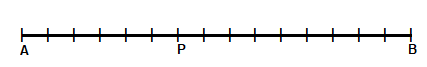P should be marked 6 cm away from A.

• Sita and Soby divided some money m the ratio 3 : 2 and Sita got 480 rupees. What is the total amount they divided?
Sita and Soby divided some money ‘m’ in the ratio 3 : 2 and Sita got 480 rupees.
Let the ratio be m, then the ratio of money Sita got will be 3m.
3m = 480
m = $$\frac{480}{3}$$
m = 160
Money Soby will get 2×160 = 320 rupees.

• In a right triangle, the two smaller angles are in the ratio 1 : 4. Compute these angles.
In a right triangle, the two smaller angles are in the ratio 1 : 4.
Right triangle means 90 degrees.
Let the ratio be x, total will be 1x + 4x = 5x.
$$\frac{1x}{5x}$$×90 = 18 degrees
$$\frac{4x}{5x}$$×90 = 72 degrees
Then the angles will be 18 degrees and 72 degrees.

• Draw a rectangle of perimeter 30 centimetres and lengths of sides in the ratio 1 : 2. Draw two more rectangles of the same perimeter, with lengths of sides in the ratio 2 : 3 and 3 : 7. Compute the areas of all three rectangles.
A rectangle of perimeter 30 centimetres is drawn with lengths of sides in the ratio 1 : 2
Let the ratio be x, the total will be 1x + 2x = 3x.
One of the side will be $$\frac{1x}{3x}$$×30 = 10 centimetres
The other side will be $$\frac{2x}{3x}$$×30 = 20 centimetres
Therefore, the rectangle with 10 centimetres and 20 centimetres is drawn as shown below.
Area of this rectangle will be 10×5=50 square centimetres.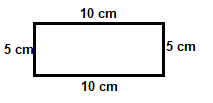A rectangle of perimeter 30 centimetres is drawn with lengths of sides in the ratio 2 : 3
Let the ratio be x, the total will be 2x + 3x = 5x.
One of the side will be $$\frac{2x}{5x}$$×30 = 12 centimetres
The other side will be $$\frac{3x}{5x}$$×30 = 18 centimetres
Therefore, the rectangle with 12 centimetres and 18 centimetres is drawn as shown below.
Area of this rectangle will be 9×6=54 square centimetres.A rectangle of perimeter 30 centimetres is drawn with lengths of sides in the ratio 3 : 7
Let the ratio be x, the total will be 3x + 7x = 10x.
One of the side will be $$\frac{3x}{10x}$$×30 = 9 centimetres
The other side will be $$\frac{7x}{10x}$$×30 = 21 centimetres
Therefore, the rectangle with 9 centimetres and 21 centimetres is drawn as shown below.
Area of this rectangle will be 10.5×4.5=47.25 square centimetres.